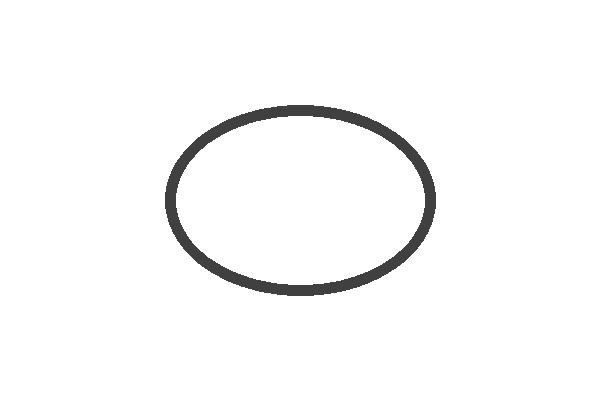# How to draw an ellipse in OpenCV using Java?

The org.opencv.imgproc package of Java OpenCV library contains a class named Imgproc. To draw an ellipse you need to invoke the ellipse() method of this class. This method accepts the following parameters −

• A Mat object representing the image on which the ellipse is to be drawn.

• A RotatedRect object (The ellipse is drawn inscribed in this rectangle.)

• A Scalar object representing the color of the Rectangle(BGR).

• An integer representing the thickness of the Rectangle(default:1).

## Example

import org.opencv.core.Core;
import org.opencv.core.Mat;
import org.opencv.core.Point;
import org.opencv.core.RotatedRect;
import org.opencv.core.Scalar;
import org.opencv.core.Size;
import org.opencv.highgui.HighGui;
import org.opencv.imgcodecs.Imgcodecs;
import org.opencv.imgproc.Imgproc;
public class DrawingEllipse {
public static void main(String args[]) {
//Reading the source image in to a Mat object
//Drawing an Ellipse
RotatedRect box = new RotatedRect(new Point(300, 200), new Size(260, 180), 180);
Scalar color = new Scalar(64, 64, 64);
int thickness = 10;
Imgproc.ellipse (src, box, color, thickness);
//Saving and displaying the image
Imgcodecs.imwrite("arrowed_line.jpg", src);
HighGui.imshow("Drawing an ellipse", src);
HighGui.waitKey();
}
}

## Output

On executing, the above program generates the following window −